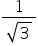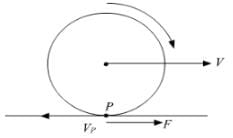NEET  >  Test: Friction

# Test: Friction

Test Description

## 10 Questions MCQ Test Physics Class 11 | Test: Friction

Test: Friction for NEET 2023 is part of Physics Class 11 preparation. The Test: Friction questions and answers have been prepared according to the NEET exam syllabus.The Test: Friction MCQs are made for NEET 2023 Exam. Find important definitions, questions, notes, meanings, examples, exercises, MCQs and online tests for Test: Friction below.
Solutions of Test: Friction questions in English are available as part of our Physics Class 11 for NEET & Test: Friction solutions in Hindi for Physics Class 11 course. Download more important topics, notes, lectures and mock test series for NEET Exam by signing up for free. Attempt Test: Friction | 10 questions in 10 minutes | Mock test for NEET preparation | Free important questions MCQ to study Physics Class 11 for NEET Exam | Download free PDF with solutions
 1 Crore+ students have signed up on EduRev. Have you?
Test: Friction - Question 1

### Complete the sentence. Friction always ____________

Detailed Solution for Test: Friction - Question 1

Frictional forces act over the common surfaces of the two bodies to avoid and restrict relative moment between those two surfaces.

Test: Friction - Question 2

### After the body starts moving, the friction involved with motion is

Detailed Solution for Test: Friction - Question 2

When the body is in rest it is under static friction but when it starts moving (neither rolling nor sliding), the static friction slowly chngs to kinetic friction as the coefficient of static friction start decreasing and that of kinetic friction starts increasing. In case it starts rolling motion then the friction is rolling friction & if it slides then sliding fiction.

Test: Friction - Question 3

### Impending motion of a body is opposed by

Detailed Solution for Test: Friction - Question 3

The impending motion refers to the state of a body when it is on the verge of slipping. In such cases, the static friction has reached its upper limit and is given by the equation, F=μsN

Test: Friction - Question 4

A block of mass 1 kg lies on a horizontal surface in a truck. The coefficient of static friction between the block and the surface is 0.6. If the acceleration of the truck is 5 ms-2, the frictional force acting on the block is

Detailed Solution for Test: Friction - Question 4

Limiting friction, f = μmg = 0.6 × 1 × 9.8 = 5.88 N

Applied force = F = ma = 1 × 5 = 5 N

As F < f, so force of friction = 5 N

Test: Friction - Question 5

A particle moves in a circle with a constant speed of 6m /s. Its centripetal acceleration is 120 m/s2, The radius of the circle is

Detailed Solution for Test: Friction - Question 5

We know that centripetal acceleration, c = v2/r
Thus we get r = v2/c
= 36 / 120
= .3 /m

Test: Friction - Question 6

A body rests on an inclined plane and the angle of inclination is varied till the body just begins to slide down. The coefficient of friction is. What is the angle of inclination?

Detailed Solution for Test: Friction - Question 6

At the time when the block just starts to move, we get that net force acting upon it is 0, thus we get, f - mg.sin a = 0
Where f is friction force and a is angle of incline.
We also know that f = 1√3 x N
= 1√3 x mg.cos a
Thus we get 1√3 x mg.cos a = mg.sin a
Thus we get tan a = 1√3
And a = 30

Test: Friction - Question 7

A block of mass 2kg rests on a plane inclined at an angle of 30with the horizontal. The coefficient of friction between the block and the surface is 0.7. The frictional force acting on the block is

Detailed Solution for Test: Friction - Question 7

Mass of the block = 2 kg

Weight of the block = mg = 2 × 9.8 = 19.6 N

The component of the weight along normal = 19.6 Cos 30° = 16.97 N

Hence, the normal force (N) = 16. 97 N

Now, the component of the weight along the inclined plane = 19.6 Sin 30°

Along the inclined plane = 9.8 N

Now, the friction = μ N = 0.7 × 16.97 = 11.87 N

Since, 9.8 N is less than the maximum value of the friction (11.87 N)

Hence, the frictional force = 9.8 N

Test: Friction - Question 8

A particle moves in a circle of radius 0.30m with a constant speed of 6m/s. Its centripetal acceleration is

Detailed Solution for Test: Friction - Question 8

We know that centripetal acceleration, a = v x v /r
Here v = 6m/s and r = 0.3m
Thus a = 36 / 0.3 m/s2
= 120 m/s2

Test: Friction - Question 9

When a wheel rolls on a level road, the direction of frictional force at the point of contact of wheel and ground is

Detailed Solution for Test: Friction - Question 9What you need to remember and apply here is that friction always tries to oppose motion.
So, in case of the wheel, the point P which is in contact with the ground tries to go backwards due to rotation and it's velocity as shown in the figure.
The frictional force F will try to oppose this motion and thus, it will be in the forward direction.

Test: Friction - Question 10

A force of 20 N is applied on a body of mass 4 kg kept on a rough surface having coefficient of friction 0.1. Find acceleration of body. Take g = 10 m/s2

Detailed Solution for Test: Friction - Question 10

The normal force due to the weight of the bloch is 40N, hence the friction force is 4N acting to restrict the motion.
Hence the net force on the body is thus 20 - 4 = 16N
Thus the net acceleration of the block is 16/4 = 4ms2

## Physics Class 11

127 videos|464 docs|210 tests
 Use Code STAYHOME200 and get INR 200 additional OFF Use Coupon Code